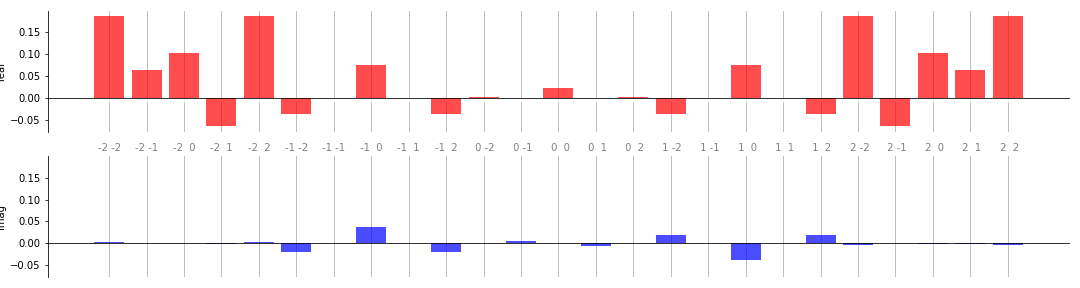# qml.fourier.visualize.bar¶

bar(coeffs, n_inputs, ax, colour_dict=None, show_freqs=True)[source]

Plot a set of Fourier coefficients as a bar plot.

Parameters
• coeffs (array[complex]) – A single set of Fourier coefficients. The dimensions of the coefficient array should be (2d + 1, ) * n_inputs where d is the largest frequency.

• n_inputs (int) – The number of input variables in the function.

• ax (list[matplotlib.axes.Axes]) – Axis on which to plot. Must be a pair of axes from a subplot where sharex="row" and sharey="col".

• colour_dict (dict[str, str]) – A dictionary of the form {"real" : colour_string, "imag" : other_colour_string} indicating which colours should be used in the plot.

• show_freqs – Whether or not to print the frequency labels on the plot axis.

Example

Suppose we have the following quantum function:

dev = qml.device('default.qubit', wires=2)

@qml.qnode(dev)
def circuit_with_weights(w, x):
qml.RX(x, wires=0)
qml.RY(x, wires=1)
qml.CNOT(wires=[1, 0])

qml.Rot(*w, wires=0)
qml.Rot(*w, wires=1)
qml.CNOT(wires=[1, 0])

qml.RX(x, wires=0)
qml.RY(x, wires=1)
qml.CNOT(wires=[1, 0])

return qml.expval(qml.PauliZ(0))


We would like to compute and plot a single set of Fourier coefficients. We will choose some values for w at random:

from functools import partial

n_inputs = 2
degree = 2

weights = np.random.normal(0, 1, size=(2, 3))
coeffs = coefficients(partial(circuit_with_weights, weights), n_inputs, degree)


We can now plot by setting up a pair of matplotlib axes and passing them to the plotting function:

>>> import matplotlib.pyplot as plt
>>> from pennylane.fourier.visualize import bar
>>> fig, ax = plt.subplots(2, 1, sharey=True, figsize=(15, 4))
>>> bar(coeffs, n_inputs, ax, colour_dict={"real" : "red", "imag" : "blue"})